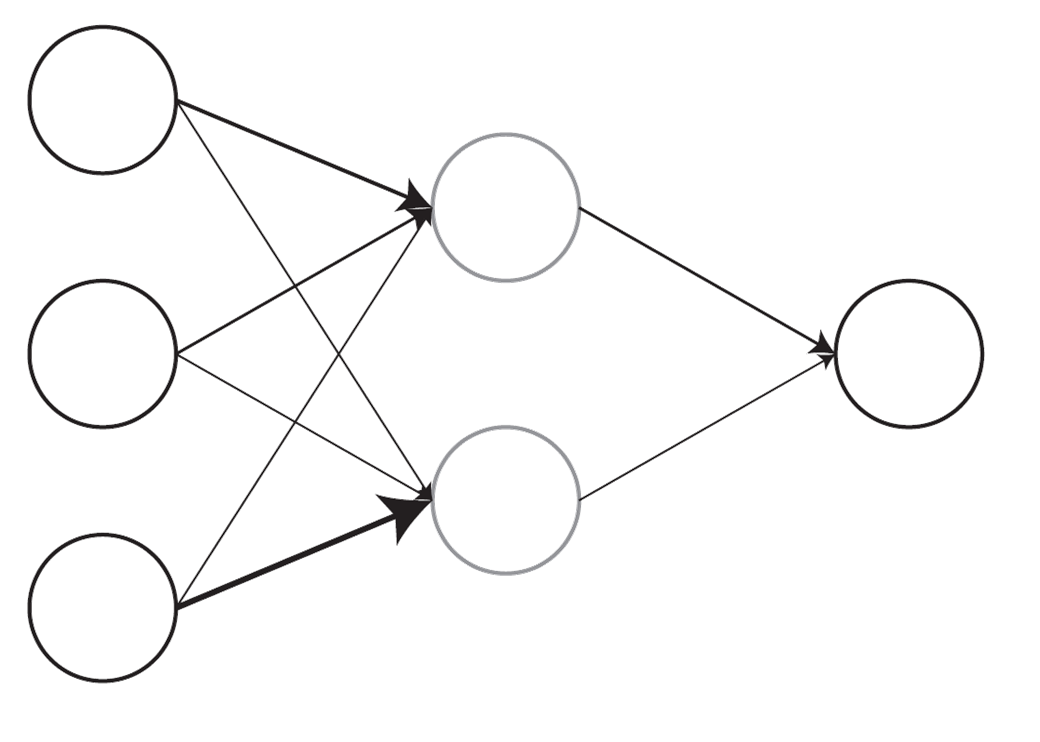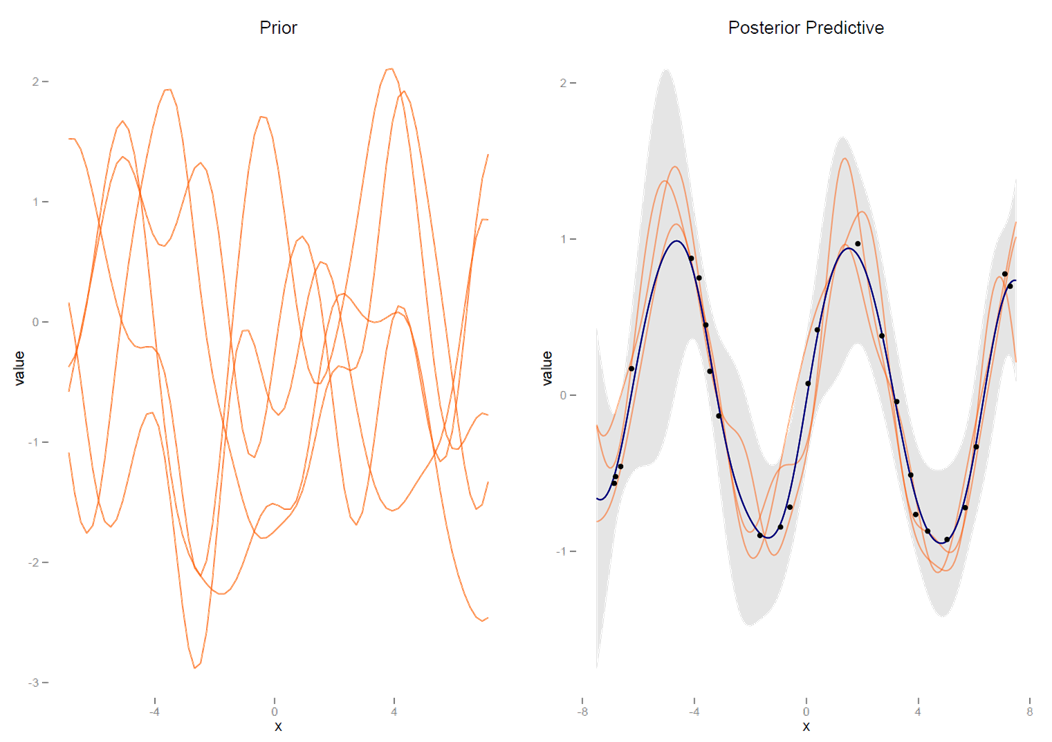# Other Approaches

This section will discuss some ways to relate GAMs to other forms of nonlinear modeling approaches, some familiar and others perhaps less so. In addition, I will note some extensions to GAMs to consider.

## Other Nonlinear Modeling Approaches

### Known Functional Form

A general form for linear and nonlinear models: $y = f(X,\beta)+\epsilon$It should be noted that one can place generalized additive models under a general heading of nonlinear models whose focus may be on transformations of the outcome (as with generalized linear models), the predictor variables (polynomial regression and GAMs), or both (GAMs), in addition to those whose effects are nonlinear in the parameters26. A primary difference between GAMs and those models is that we don’t specify the functional form beforehand with GAMs.

In cases where the functional form may be known, one can use an approach such as nonlinear least squares, and there is inherent functionality within a standard R installation, such as the nls function. As is the usual case, such functionality is readily extendable to a great many other analytic situations, e.g. the gnm for generalized nonlinear models or nlme for nonlinear mixed effects models.

### Response Transformation

As noted, it is common practice, perhaps too common, to manually transform the response and go about things with a typical linear model. While there might be specific reasons for doing so, the primary reason applied researchers seem to do so is to make the distribution ‘more normal’ so that regular regression methods can be applied, which stems from a misunderstanding of the assumptions of standard regression. As an example, a typical transformation is to take the log, particularly to tame ‘outliers’ or deal with heteroscedasticity.

While it was a convenience ‘back in the day’ because we didn’t have software or computing power to deal with a lot of data situations aptly, this is definitely not the case now. In many situations, it would be better to, for example, conduct a generalized linear model with a log link or perhaps assume a different distribution for the response directly (e.g. log- or skew-normal), and many tools allow researchers to do this with easeA lot of ‘outliers’ tend to magically go away with an appropriate choice of distribution for the data generating process..

There are still cases where one might focus on response transformation, just not so one can overcome some particular nuisance in trying to fit a linear regression. An example might be in some forms of functional data analysis, where we are concerned with some function of the response that has been measured on many occasions over time. Another example would be in economics where one wishes to speak in terms of elasticities.

### The Black Box

Venables and Ripley (2002, Section 11.5) make an interesting delineation of nonlinear models into those that are less flexible but under full user control (fully parametric)One could probably make the case that most modeling is ‘black box’ for a great many researchers., and those that are black box techniques that are highly flexible and fully automatic: stuff goes in, stuff comes out, but we’re not privy to the specifics27.

Two examples of the latter that they provide are projection pursuit and neural net models, though a great many would fall into such a heading. Projection pursuit models are well suited to high dimensional data where dimension reduction is a concern. One may think of an example where one uses a technique such as principal components analysis on the predictor set and then examines smooth functions of $$M$$ principal components.

In the case of neural net modelsA Neural Net Model

, which have found a resurgence of interest of late to say the least under the heading of deep learning, one can imagine a model where the input units (predictor variables) are weighted and summed to create hidden layer units, which are then transformed and put through the same process to create outputs (see a simple example to the right). One can see projection pursuit models as an example where a smooth function is taken of the components which make up the hidden layer. Neural networks are highly flexible in that there can be any number of inputs, hidden layers, and outputs. And, while such models are very explicit in the black box approach, tools for interpretability have been much more accessible these days.

Such models are usually found among machine learning techniques, any number of which might be utilized in a number of disciplines. Other more algorithmic/black box approaches include networks/graphical models, random forests, support vector machines, and various tweaks or variations thereof including boosting, bagging, bragging and other alliterative shenanigans28. As Venables and Ripley note, generalized additive models might be thought of as falling somewhere in between the fully parametric and highly interpretable models of linear regression and more black box techniques. Indeed, there are even algorithmic approaches which utilize GAMs as part of their approach.

## Extensions

### Other GAMs

#### Categorical variables

Note that just as generalized additive models are an extension of the generalized linear model, there are generalizations of the basic GAM beyond the settings described. In particular, random effects can be dealt with in this context as they can with linear and generalized linear models, and there is an interesting connection between smooths and random effects in general.29 This allowance for categorical variables, i.e. factors, works also to allow separate smooths for each level of the factor. This amounts to an interaction of the sort we demonstrated with two continuous variables. See the appendix for details.

#### Spatial Modeling

Additive models also provide a framework for dealing with spatially correlated data as well. As an example, a Markov Random Field smooth can be implemented for discrete spatial structure, such as countries or statesIncidentally, this same approach would potentially be applicable to network data as well, e.g. social networks.. For the continuous spatial domain, one can use the 2d smooth as was demonstrated previously, e.g. with latitude and longitude. Again one can consult the appendix for demonstration, and see also the Gaussian process paragraph.

#### Structured Additive Regression Models

The combination of random effects, spatial effects, etc. into the additive modeling framework is sometimes given a name of its own- structured additive regression models, or STARs30. It is the penalized regression approach that makes this possible, where we have a design matrix that might include basis functions or an indicator matrix for groups, and an appropriate penalty matrix. With those two components, we can specify the models in almost identical fashion, and combine such effects within a single model. This results in a very powerful regression modeling strategy. Furthermore, the penalized regression described has a connection to Bayesian regression with a normal, zero-mean prior for the coefficients, providing a path toward even more flexible modelingThe brms package serves as an easy to use starting point in R, and has functionality for using the mgcv package’s syntax style..

#### GAMLSS

Generalized additive models for location, scale, and shape (GAMLSS) allow for distributions beyond the exponential family31, and modeling different parameters besides the mean. mgcv has recently added several options in this regard as well.

#### Other

In addition, there are boosted, ensemble and other machine learning approaches that apply GAMs. See the GAMens package for example. Also, boosted models are GAMs. In short, there’s plenty to continue to explore once one gets the hang of generalized additive models.

### Reproducing Kernel Hilbert Space

Generalized smoothing splines are built on the theory of reproducing kernel Hilbert spaces. I won’t pretend to be able to get into it here, but the idea is that some forms of additive models can be represented in the inner product form used in RKHS approaches32. This connection lends itself to a tie between GAMs and e.g. support vector machines and similar methods. For the interested, I have an example of RKHS regression here.

### Gaussian Processes

We can also approach modeling by using generalizations of the Gaussian distribution. Where the Gaussian distribution is over vectors and defined by a mean vector and covariance matrix, a Gaussian Process is a distribution over functions. A function $$f$$ is distributed as a Gaussian Process defined by a mean function $$m$$ and covariance function $$k$$. They have a close tie to RKHS methods, and generalize commonly used models in spatial modeling.Gaussian Process
The left graph shows functions from the prior distribution, the right shows the posterior mean function, 95% confidence interval shaded, as well as specific draws from the posterior predictive mean distribution.

$f\sim \mathcal{GP}(m,k)$

In the Bayesian context, we can define a prior distribution over functions and make draws from a posterior predictive distribution of $$f$$ once we have observed data. The reader is encouraged to consult Rasmussen and Williams (2006) for the necessary detail. The text is free for download, and Rasmussen also provides a nice and brief intro. I also have some R code for demonstration based on his Matlab code, as well as Bayesian examples in Stan.

Suffice it to say in this context, it turns out that generalized additive models with a tensor product or cubic spline smooth are maximum a posteriori (MAP) estimates of Gaussian processes with specific covariance functions and a zero mean function. In that sense, one might segue nicely to Gaussian processes if familiar with additive models. The mgcv package also allows one to use a spline form of Gaussian process.

### References

Venables, William N., and Brian D. Ripley. 2002. Modern Applied Statistics with S. Birkhäuser.

Rasmussen, Carl Edward, and Christopher K. I Williams. 2006. Gaussian Processes for Machine Learning. Cambridge, Mass.: MIT Press.

1. For example, various theoretically motivated models in economics and ecology. A common model example is the logistic growth curve.

2. For an excellent discussion of these different approaches to understanding data see Breiman (2001) and associated commentary. For some general packages outside of R that incorporate a more algorithmic approach to modeling, you might check out the scikit-learn module for Python as a starting point.

3. See Hastie, Tibshirani, and Friedman (2009) for an overview of such approaches. A more recent and very applied version of that text is also available. I have an R oriented intro here.

4. Wood (2017) has a whole chapter devoted to the subject, though Fahrmeir et al. (2013) provides an even fuller treatment. I also have a document on mixed models that goes into it some. In addition, Wood also provides a complementary package, gamm4, for adding random effects to GAMs via lme4.

5. Linkedin has used what they call GAME, or Generalized Additive Mixed-Effect Model, though these are called GAMMs (generalized additive mixed model) practically everywhere else. The GAME implementation does appear to go beyond what one would do with the gamm function in mgcv, or at least, takes a different and more scalable computational approach.

6. See Rigby and Stasinopoulos (2005) and the gamlss package.

7. You might note that the function used in the spline example in the technical section is called rk.